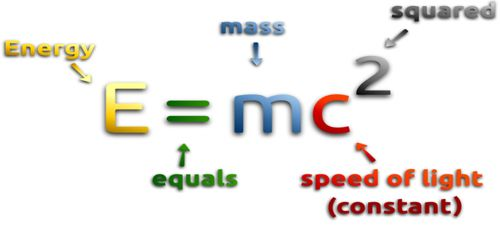Physics

# Mass-Energy RelationMass-energy relation: According to the special theory of relativity, the relation between mass and energy is E = mc2, here E = energy, m = mass of the object and c = the speed of light. Energy is the function of mass. The bigger a mass is the bigger amount of energy it will gain or release.

Einstein used E = mc2 to prove that mass and energy are relative to each other. The equation E = mc2 states that the amount of energy possessed by an object is equal to its mass multiplied by the square of the speed of light. Since the speed of light is an extremely high number, almost 300,000 km/sec, a small amount of mass contains a lot of energy. This formula states that the equivalent energy (E) can be calculated as the mass (m) multiplied by the speed of light (c = about 3×108 m/s) squared. Additionally, the equation suggests that energy and mass are transposable with each other. In other words, energy can be converted into mass and mass to energy. Mass and energy are equivalent and are connected mathematically by Einstein’s mass-energy relation.